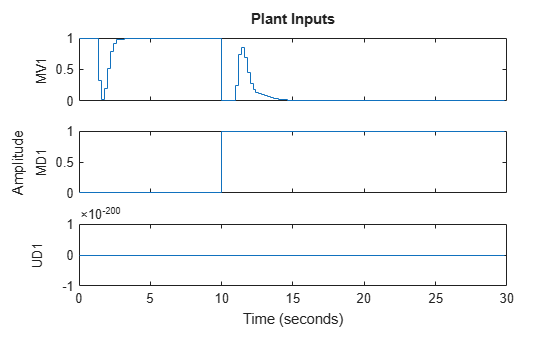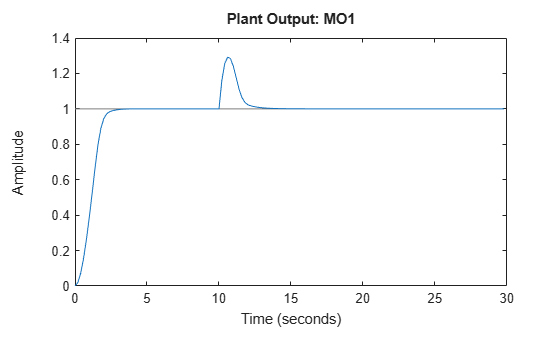# sim

Simulate an MPC controller in closed loop with a linear plant

## Syntax

``sim(mpcobj,Ns,r)``
``sim(mpcobj,Ns,r,v)``
``sim(___,SimOptions)``
``[y,t,u,xp,xc,SimOptions] = sim(___)``

## Description

Use the Model Predictive Control Toolbox™ `sim` function to simulate, in discrete time, the closed-loop or open-loop response of a plant and an MPC controller with constraints and weights that do not change at run time. The MPC controller can be implicit or explicit, the controlled plant must be linear and time-invariant, and you must specify the reference and disturbance signals in advance. By default, the plant used in the simulation is the one in `MPCobj.Model.Plant`, but you can use a different plant model to assess the controller robustness to model mismatch.

To run simulink models programmatically instead, see `sim` (Simulink).

````sim(mpcobj,Ns,r)` simulates the closed-loop response to the specified reference signal, `r`. The simulation runs in discrete time, with sample time `mpcobj`, for `Ns-1` simulation steps, and simulation results are plotted. The plant model is the one specified in `mpcobj.Model.Plant` (which is discretized or resampled, if needed). The MPC controller `mpcobj` can be either a traditional MPC controller (`mpc`) or explicit MPC controller (`explicitMPC`). If you omit `Ns` or `r` then default values are used.```

example

````sim(mpcobj,Ns,r,v)` also specifies the measured disturbance signal `v`. ```
````sim(___,SimOptions)` specifies additional simulation options. This syntax allows you to alter the default simulation options, such as initial states, input/output noise, and unmeasured disturbances, plant mismatch, etc. It also allows you to simulate the plant in open loop. You can use `SimOptions` with any of the previous input combinations.```
````[y,t,u,xp,xc,SimOptions] = sim(___)` suppresses plotting and instead returns: the sequence of plant outputs `y`,the time sequence `t` (equally spaced by `mpcobj.Ts`),the manipulated variables `u` generated by the MPC controller,the sequence `xp` of states of the model of the plant used for simulation,the sequence `xmpc` of states of the MPC controller (provided by the state observer),and the simulation options object, `SimOptions`. ```

## Examples

collapse all

Simulate the MPC control of a MISO system. The system has one manipulated variable, one measured disturbance, one unmeasured disturbance, and one output.

Create the continuous-time plant model. This plant will be used as the prediction model for the MPC controller.

`sys = ss(tf({1,1,1},{[1 .5 1],[1 1],[.7 .5 1]}));`

Discretize the plant model using a sampling time of 0.2 units.

```Ts = 0.2; sysd = c2d(sys,Ts);```

Specify the MPC signal type for the plant input signals.

`sysd = setmpcsignals(sysd,'MV',1,'MD',2,'UD',3);`

Create an MPC controller for the `sysd` plant model. Use default values for the weights and horizons.

`MPCobj = mpc(sysd);`
```-->The "PredictionHorizon" property is empty. Assuming default 10. -->The "ControlHorizon" property is empty. Assuming default 2. -->The "Weights.ManipulatedVariables" property is empty. Assuming default 0.00000. -->The "Weights.ManipulatedVariablesRate" property is empty. Assuming default 0.10000. -->The "Weights.OutputVariables" property is empty. Assuming default 1.00000. ```

Constrain the manipulated variable to the `[0 1]` range.

`MPCobj.MV = struct('Min',0,'Max',1);`

Specify the simulation stop time.

`Tstop = 30;`

Define the reference signal and the measured disturbance signal.

```num_sim_steps = round(Tstop/Ts); r = ones(num_sim_steps,1); v = [zeros(num_sim_steps/3,1); ones(2*num_sim_steps/3,1)];```

The reference signal, `r`, is a unit step. The measured disturbance signal, `v`, is a unit step, with a 10 unit delay.

Simulate the controller.

`sim(MPCobj,num_sim_steps,r,v)`
```-->The "Model.Disturbance" property is empty: Assuming unmeasured input disturbance #3 is integrated white noise. Assuming no disturbance added to measured output channel #1. -->The "Model.Noise" property is empty. Assuming white noise on each measured output. ```## Input Arguments

collapse all

Model predictive controller, specified as one of the following:

Number of simulation points, including zero, specified as a positive integer. The simulation runs for `Ns-1` steps, between `0` and `Ts*(Ns-1)`.

If you omit `Ns`, the default value is the number of rows of whichever of the following arrays has the largest number of rows:

• The input argument `r`

• The input argument `v`

• The `UnmeasuredDisturbance` property of `SimOptions`, if specified

• The `OutputNoise` property of `SimOptions`, if specified

• The greatest value between the prediction horizon `MPCobj.P` and `10`.

Example: `20`

Reference signal, specified as an array. This array has `ny` columns, where `ny` is the number of total (measured and unmeasured) plant outputs. `r` can have anywhere from 1 to `Ns` rows. If the number of rows is less than `Ns`, the missing rows are set equal to the last row.

If Ns is empty or unspecified, it defaults to the nominal output vector `MPCobj.Model.Nominal.Y`.

Example: `ones(20,3)`

Measured input disturbance signal, specified as an array. This array has `nv` columns, where `nv` is the number of measured input disturbances. `v` can have anywhere from 1 to `Ns` rows. If the number of rows is less than `Ns`, the missing rows are set equal to the last row.

If `v` is empty or unspecified, it defaults to the nominal value of the measured input disturbance, `MPCobj.Model.Nominal.U(md)`, where `md` is the vector containing the indices of the measured disturbance signals, as defined by `setmpcsignals`.

Example: `[zeros(50,1);ones(50,1)]`

Simulation options, used to specify additional simulation options as well as noise and disturbance signals that feed into the plant but are unknown to the controller. You can also use this object to simulate the plant in open loop, or to specify a plant model to be used in simulation that is different from the one in `MPCobj.Model.Plant`, which allows you to assess the robustness of the control loop response to model mismatch.

For more information, see `mpcsimopt`.

## Output Arguments

collapse all

Sequence of plant outputs values, returned as a `Ns`-by-`Ny` array, where `Ns` is the number of simulation steps and `Ny` is the number of plant outputs. The values in `y` include neither additive output disturbances nor additive measurement noise (if any).

Time sequence, returned as a `Ns`-by-1 array, where `Ns` is the number of simulation steps. The values in `t` are equally spaced by `MPCobj.Ts`.

Sequence of manipulated variables values generated by the MPC controller, returned as a `Ns`-by-`Nu` array, where `Ns` is the number of simulation steps and `Nu` is the number of manipulated variables.

Sequence of plant model states values, returned as an `Ns`-by-`Nxp` array, where `Ns` is the number of simulation steps and `Nxp` is the number of states in the plant model. The plant model is either `MPCobj.Model` or `SimOptions.Model`, if the latter is specified.

Sequence of MPC controller states, returned as an `Ns`-by-1 structure array. Each entry in the structure array has the same fields as an `mpcstate` object. The controller uses a built-in linear Kalman filter to estimate the state of the plant, augmented by the disturbance and noise models. The state of the controller is the state of its internal Kalman filter. For open-loop simulations, `xc` is empty.

Simulation options objects used for the simulation. This object can specify noise and disturbance signals that feed into the plant but are unknown to the controller. It can also specify if the simulated system is open loop or if the plant model used in the simulation is different from the one in `MPCobj.Model.Plant`.

For more information, see `mpcsimopt`.

## Version History

Introduced before R2006a